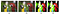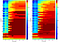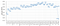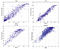# Reading: DeepSim — Deep Similarity for (Image Quality Assessment)

## Using ImageNet Pretrained VGGNet, Outperforms Handcrafted-Feature IQA: MSSIM, FSIM, GMSD & RMSE

this story, “DeepSim: Deep similarity for image quality assessment” (DeepSim), by Hangzhou Dianzi University, Zhejiang University City College, and Zhejiang University of Technology, is presented. I read this paper because recently I need to study/work on IQA/VQA (Image Quality Assessment/Video Quality Assessment). In this story:

• First, the features of the reference and tested images are obtained by ImageNet pretrained VGGNet without any further training.
• Then, the local similarities between the features are measured.
• Finally, the local quality indices are gradually pooled together to estimate the overall quality score.

This is a paper in 2017 JNEUCOM (Neurocomputing) with over 50 citations where JNEUCOM is a journal in Elsevier with high impact factor of 4.438.

# Outline

1. IQA Algorithms
2. DeepSim: Deep Similarity
3. Experimental Results

# 1. IQA Algorithms

## 1.1. The Use of IQA

• There are various aspects, such as the weak light in the environment, the improper operations of human, or the high compression rate in the transmission system, will make the obtained images poor in term of quality.

Image quality assessment (IQA) algorithms is to precisely and automatically estimate human perceived image quality.

• IQA algorithms can be embedded into cameras, mobile phones, or SNS systems to make them “intelligent” enough to automatically capture, enhance, process, or display images optimally.

## 1.2. FR/RR/NR-IQA

• FR-IQA methods are always applied in the laboratory environments since the reference image is needed where it is usually absent in real application.
• RR-IQA methods are suitable for the network transmission scenarios.
• NR-IQA methods are suitable for most practical applications where it is impossible or of great difficulty to obtain the reference image.

Traditionally, both the features and the mapping function are hand-crafted.
(At that moment, there are not much deep learning approaches.)

DeepSim is FR-IQA using VGGNet.

# 2. DeepSim: Deep Similarity

## 2.1. VGGNet

• The above is the VGGNet network architecture.
• At that moment, ReLU and max pooling layer is also counted as layer. Thus, it is mentioned that this network has 37 layers.
• Indeed, it is VGG-16, i.e. VGGNet with 16 weight layers.

ImageNet pre-trained VGG-16 is used in DeepSim without any further training or fine-tuning.

## 2.2. DeepSim Using VGGNet

• The kth feature map in the lth layer derived from the reference image and the test image are denoted by F(r)l,k and F(t)l,k, with k=1, 2, …, Kl and l=1, 2, …, L, respectively, where L=37 is the total number of layers.

## 2.3. Local Similarity Measure

• Suppose x = {xi | i = 1 , 2 , . . . , n } be the d×d area centered at location (i, j) in F(r)l,k, and y = {yi | i = 1 , 2 , . . . , n } the relevant area in F(t)l,k.
• And n=d×d, which is the number of elements within d×d.
• Gaussian filter w = { wi | i = 1 , 2 , . . . , n }, with standard deviation of 1.5, is applied to estimate local statistics:
• Then, the similarity index associated with location (i, j) is estimated:
• where Ml,k denote the quality map related to the kth feature map in the lth layer, k=1, 2, …, Kl and l=1, 2, …, L.
• In this paper, d = 11, C1 = βfl,k and C2 = βfl,k, with β1 = 0.01 , β2 = 0.03 , fl,k is the maximium magnitude of the feature values over both F(r)l,k and F(t)l,k. And it is found that the final IQA performance is insensitive to the choice of these parameters.

## 2.4. Quality Pooling

• There are 3 stages.
• Stage 1: First, local similarities in each quality map are pooled to a scalar score. In this way, we obtain Kl quality scores over the l-th layer:
• Since each feature map corresponds to one particular filter, this integrated quality score obtained here can be treated as filter-level quality.
• Stage 2: Afterwards, the filter-level qualities in each layer are pooled together to obtain the layer-level quality indices:
• Stage 3: Eventually, the L layer-level quality indices are pooled together to obtain the final global quality score.
• To perform pooling, there are 5 choices to considered: average (AVG) pooling, standard deviation (SD) pooling, mean absolute deviation (MAD) pooling, full deviation (FD) pooling, and percentile (Pp) pooling.
• 1. Average (AVG) pooling: the AVG pooling strategy adopts the mean value of all the local quality scores in q:
• 2. Percentile (Pp) pooling: The artefacts caused by distortion rather than the normal regions primarily contribute to humans’ judgments of quality. It is therefore rational to take a piece of the lowest quality indices to estimate the overall quality perception.
• First, sort q in ascending order as q(sort). Then, average the first M q(sort)i according to p% value.
• When p = 100 , it is actually the average (AVG) pooling.
• 3. Standard deviation (SD) pooling: Intuitively, by SD pooling, it means to utilize the standard deviation of the given quality indices as the final quality measure.
• 4. Mean absolute deviation (MAD) pooling: is to compute the mean absolute difference between the given local quality indices and qAVG:
• 5. Full deviation (FD) pooling: the FD pooling strategy is to combine the SD and MAD indices by:
• where 0<α<1, and α=0.5 in the paper.

# 3. Experimental Results

• Four IQA databases are tested: CSIQ, LIVE, LIVEMD, and TID2013.
• PLCC, SRCC and KRCC are evaluated. With all having similar trend, only PLCC is reported.
• avg: Overall performance across the databases.
• wgt.avg: Weighted average performance across the databases according to the number of images.

## 3.1. Preprocessing

• Preprocessing (PreProc): Rach image fed into VGGNet is resized to 224 pixels and subtracting the mean training image from each pixel. DeepSim run in this way is denoted by DeepSimPreProc.
• Original: Rhe mean training image is first resized to the same size as the input image and then subtracted from it, and is denoted by DeepSimOriginal.
• It is shown that resizing the input image before input it into VGGNet improves the quality prediction accuracy.
• Using the resized image make DeepSim insensitive to the pooling strategy. Namely, DeepSimPreProc is always highly consistent with human perception no matter which pooling strategy is adopted.
• Although DeepSimOriginal slightly outperforms DeepSimPreProc on the CSIQ, LIVE, and LIVEMD databases, DeepSimPreProc inversely show great advantage over DeepSimOriginal on TID2013 — the largest IQA database.Illustration of preprocessed images. (a) Reference image, 512 ×768 pixels; (b) JPEG20 0 0 compressed version of (a), 512 ×768 pixels; (c) Preprocessed version of (a), 224 ×224 pixels; (d) Preprocessed version of (b), 224 ×224 pixels.
• Obviously, for the regions where quality degradation occurs (e.g. the forehead of the red parrot), there are distinct differences between (c) and (d).
• Thus, resizing the original image might produces intensive abstractions of artefacts instead of diminishing them, and is beneficial for constructing robust and effective IQA metric.

## 3.2. LayersHeat maps of the average (left) and weighted average (right) PLCC values across the four databases related to each layer w.r.t different pooling strategies
• For the x-axis of the above figure, the pooling strategies are denoted by serial numbers, i.e. 1 — SD , 2 — MAD , 3 — FD ; 4–12 — { Pp | p = 10 , . . . , 90 } (sequentially), and 13 — AVG.
• When the percentile pooling or average pooling strategy is utilized, the average and weighted average PLCC values related to all the layers are high (greater than 0.8 mostly). This implies that all the features produced by all the layers in VGGNet are rather representative of image quality.
• However, when the SD, MAD, or FD strategy is utilized, the PLCC values related to the low and middle layers decrease greatly, while the high layers (i.e. layers 32–37) consistently produce high PLCC values. This might due to the fact that higher layers captures higher abstractions of image content, which can robustly represent image content.Weighted average PLCC values, between the quality scores predicted over each layer and MOS/DMOS, across the four databases
• In addition, as shown above, the middle layers produce the highest PLCC values, which are greater than 0.9. The mid-level features might capture intensive abstraction of the specific information related to quality degradation. Thus we can judiciously draw the conclusion that the mid-level features are most effective, while the high-level features are most robust, in term of representing image quality.
• Moreover, the PLCC values of the ReLU/mpool layers are generally higher than that of the corresponding preceding layer. In contrast, the softmax operation in the last layer significantly decreases the IQA performance.

## 3.3. Pooling Strategy

• Percentile pooling with p ≥30 and average pooling outperforms SD, MAD, and FD.
• The performance slightly increases as p increases, reaches the peak value when p = 60, and then slightly decreases when more quality indices are adopted.
• This is because, quality perception is a global behavior, i.e. human beings judge the quality of a given image based on the great mass of the image content.
• On the other hand, the regions of low quality contribute more to humans judge of quality than those of high quality. When only a small portion of the lowest quality indices.
• Adopting too many local quality indices (e.g. more than 60%) for pooling introduces noise to the estimated quality score.
• AVG is most commonly used and the PLCC related to AVG highly approaches the best performance, average (AVG) pooling is chosen as the default pooling.

## 3.4. Consistency with human perceptionScatter plots of the quality score, predicted by DeepSim with preprocessing and the average pooling strategy, vs. the MOS/DMOS
• The above scatter plots of the quality scores vs. MOSs/DMOSs on the four databases.
• DeepSim’s predicted quality scores are consistent with human quality perception.

## 3.5. Comparison with state-of-the-arts

• Inspiringly, DeepSim obtains significantly stronger results than existing algorithms across these databases.
• The weighted average SRCC of DeepSim is approximately 2% higher than previous state-of-the-art. Considering that VGGNet is not specially learned for IQA, this is a great achievement.

## 3.6. Computational complexity

• DeepSim is implemented by unoptimized MATLAB codes.
• DeepSim takes about 15.61s to compute each quality measure on an image of resolution 512×768 on a 1.8 GHz single-core PC with 4GB of RAM.

## Reference

[2017 JNeucom] [DeepSIM]
DeepSim: Deep similarity for image quality assessment

## Image Quality Assessment

[IQA-CNN] [DeepSim]

Written by